# Training awd-lstm

Hi i have done som gridsearch for awd-lstm:
-tokens produced by sentencepiece
-nb training tokens : 2.5e8
-validation set 0.5e8 tokens
-drop_mult=0.1,
-dropout_ profile is the default: input_p, output_p, weight_p, embed_p, hidden_p, out_bias = 0.25, 0.1, 0.2, 0.02, 0.15, True
-learn.fit_one_cycle(cyc_len=1, max_lr=g.lr, moms=(0.7, 0.8), wd=1e-5, pct_start=g.pct_start). Where g is the grid parameters

Here is pct-start vs max_lr: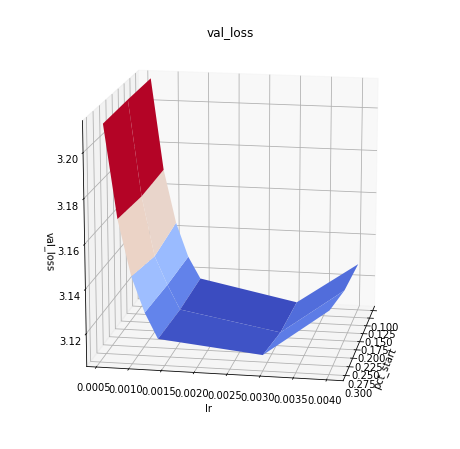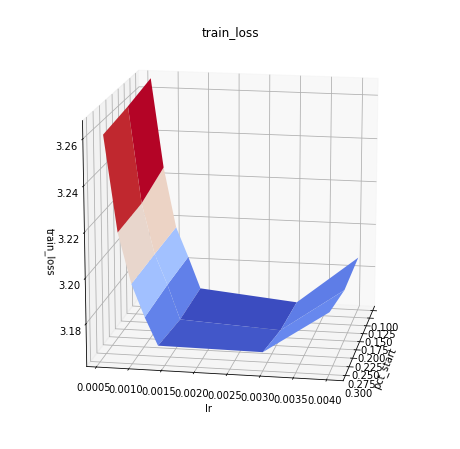The loss is lowest for:

• max_lr is in the interval 1.5e-3 to 3.5e-3
• pct_start =0.1 for the same level of max_lr. compare pct-start for the same max_lr in the following table
pct_start maxlr val_loss train_loss
0.1 0.0001 3.864176 3.9149377
0.2 0.0001 3.864224 3.9117248
0.3 0.0001 3.864385 3.9123857
0.1 0.0002 3.509694 3.5669203
0.2 0.0002 3.513564 3.5642123
0.3 0.0002 3.506579 3.5559413
0.1 0.0006 3.21159 3.2655728
0.2 0.0006 3.211637 3.262578
0.3 0.0006 3.21124 3.2605066
0.1 0.0008 3.168485 3.2242937
0.2 0.0008 3.16774 3.219342
0.3 0.0008 3.169778 3.2186317
0.1 0.001 3.143199 3.196292
0.2 0.001 3.140273 3.196489
0.3 0.001 3.144574 3.19643
0.1 0.0012 3.126952 3.1823912
0.2 0.0012 3.127207 3.1827128
0.3 0.0012 3.128621 3.1819808
0.1 0.0014 3.116634 3.1675742
0.2 0.0014 3.115873 3.1669679
0.3 0.0014 3.117083 3.1697454
0.1 0.003 3.107323 3.1631694
0.2 0.003 3.107542 3.1643822
0.3 0.003 3.112326 3.169355
0.1 0.004 3.127283 3.1858573
0.2 0.004 3.128632 3.18424
0.3 0.004 3.133826 3.188411

So the next grid search should be in the interval max_lr = 3e-3 and pct_start 0.01 to 0.1

Here is pct_start for lr_max. the trend is not clear, but having a lower pct_start (say 0.1) than the default =0.3 could be a little beneficial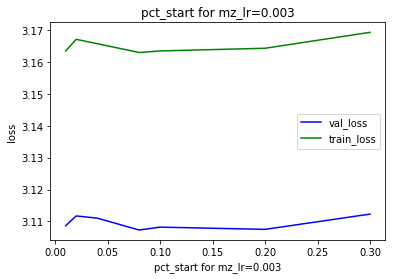and max_lr for pct_start=0.015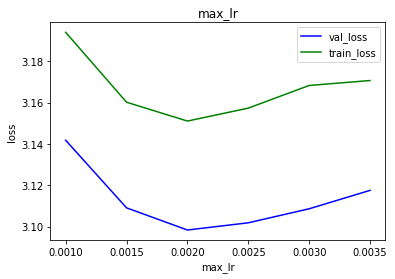Here is moms for max_lr2e-3 and pct-start=0.02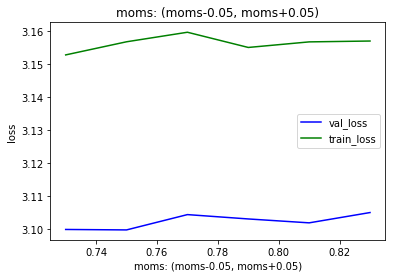The plot shows that the loss is smallest for moms = (0.7,08) which is the default for fit_one_cycle with awd_lstm. However the influence of moms is the shown range is modest. I am tempeted to use a higher moms

Here is the dependency on pct_start over a large range using :
learn.fit_one_cycle(cyc_len=1, max_lr=2e-3, moms=(0.75, 0.85), wd=1e-5, pct_start=g.pct_start)

The values:

pct_start val_loss train_loss
0 0.01 3.101077 3.1537309
1 0.02 3.105863 3.1586406
4 0.10 3.101995 3.1542885
5 0.20 3.099214 3.1598654
6 0.30 3.104660 3.1587381
2 0.40 3.109252 3.1626663
3 0.70 3.133264 3.1873376

The plot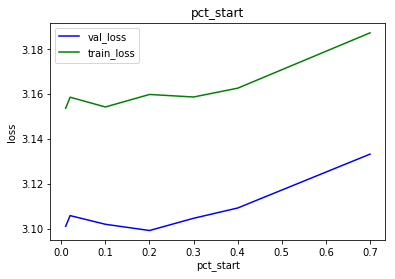This shows that the optimal pct_start is to be found in the interval 0.01 to 0.2

The following shows that the loss start to “bulk out” to the right at pct_start=0.1 and above. This is very noticeable at pct_start=0.7.
Considering that the loss is consistently smallest at pct_start<=0.2 i believe that the pattern seen at high pct_start is a sign of unhealthy training. I will therefore use pct_start=0.02 going forward:

pct_start=0.01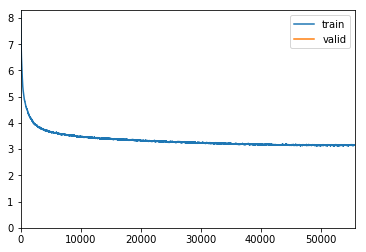pct_start=0.02pct_start=0.1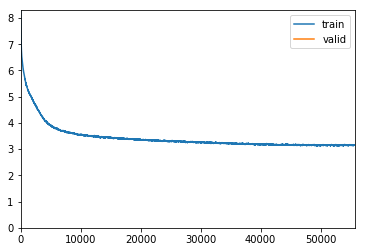pct_start=0.2pct_start=0.3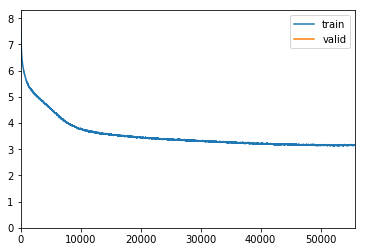pct_start=0.4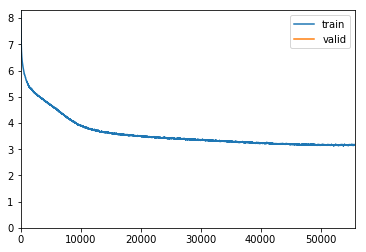pct_start=0.7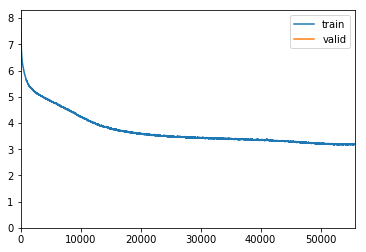Here are the results of training for 10 epoch with nbtrain tokens = 2.5e8. Training with

• fit_one_cycle(cyc_len=10 max_lr=2e-3, moms=(0.70, 0.80), wd=1e-5, pct_start=0.02) result in a validation loss of : 2.900522
• fit_one_cycle(cyc_len=epochs, max_lr=2e-3, moms=(0.70, 0.80), wd=1e-5, pct_start=0.2) result in a validation loss of : 2.888639

That is training for 1 vs 10 epochs show that pct_start=0.2 giving the lowest loss. This is probably due to the regularization effect of using a higher learning rate from the beginning